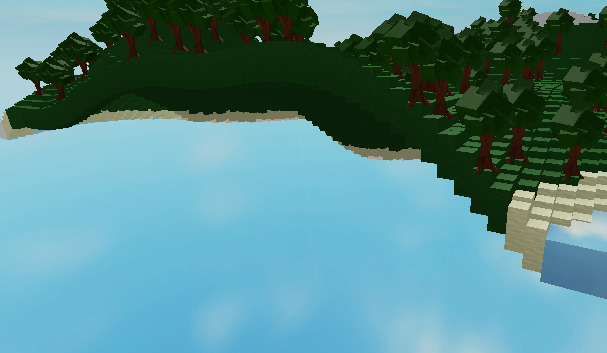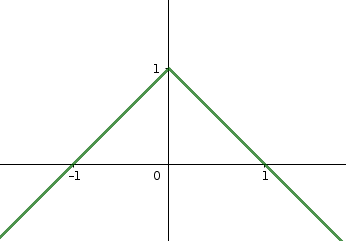# How to make the surrounding noise generation a ocean

Hello!
Im making a survival Island game which spawns you at a island in the ocean! But the problem is the generation of procedure cant be modified except a height level which the more height value. The more block level its goes! I tried to calculate the x lane and y lane then added a water if it’s just started but it still didnt work.

What i want (example):

Result I getSince I learned this by tutorial. I dont know much about noise.
I appreciate every reply if it’s related to the topic!

Thanks for reading2 Likes

One solution is to calculate the horizontal distance from each block to the center of the island, e.g. the coordinate (0, 0, 0). Then subtract that distance from the height. That way the island will be taller in the center and get lower and lower further away until it finally goes below the water line.

One improvement is to not just use the distance. Here’s a 2D from-the-side plot of how the height of the island would behave:It has a sharp corner in the middle where it suddenly stops going up and starts going down in an instant, which might look ugly in the generated map. If we use a different curve than a straight line we can get smoother results. One curve that’s pretty smooth is the smoother-step function

As you can see it starts perfectly flat at x=0 and ends up perfectly flat at x=1. If we put the distance from the center of the island in place of x then we can use that as a multiplier for the height. If we divide the distance by the radius of the island we get some control over the size of the island.

The smootherstep function is `-2 x³ + 3x²`. If we flip it and scoot it over a bit we get the function that is shown with the orange graph: `-2 (-x+1)³ + 3(-x+1)²`. Which looks like this in code:

``````function smootherStep(x)
return 2 * math.pow(x, 3) + 3 * math.pow(x, 2)
end

function smootherStep1To0(x)
return smootherStep(-x + 1)
end
``````

If you have some function for calculating the height of the terrain at a given (X, Z) coordinate, you can modify it to use this function like so:

``````local ISLAND_RADIUS = 100
function coordinateHeightMultiplier(x, z)
local dist = math.clamp( Vector3.new(x, 0, z).Magnitude, 0, ISLAND_RADIUS )
end

function coordinateHeight(x, z)
--- whatever code you have for calculating the height of the island...
-- height = height of the terrain before applying the modifier

return height * coordinateHeightMultiplier(x, z)
end
``````

If you don’t all this smootherstep stuff you can replace the multiplier function with this instead:

``````local ISLAND_RADIUS = 100
function coordinateHeightMultiplier(x, z)
local dist = math.clamp( Vector3.new(x, 0, z).Magnitude, 0, ISLAND_RADIUS )
return 1 - (dist / ISLAND_RADIUS)
end
``````
4 Likes

I got the idea what you thinking. It seems to work pretty well! But the problem is that
ISLAND [Beta Feature] - Roblox Studio (gyazo.com)
The Vector 0,0 part isnt a middle part. Instead it’s a first part that spawns!
So, I changed the
` local dist = math.clamp( Vector3.new(x, 0, z).Magnitude, 0, ISLAND_RADIUS )`

and change the magnitude by letting a middle position of the map, which can be found by letting x and z be a map size and divide by 2. Which is a middle position. Then, I subtract the current block x and z by the middle of the map. The result is looking like this:
ISLAND [Beta Feature] - Roblox Studio (gyazo.com)

Thank you for helping me!

Final result of modifying:
ISLAND [Beta Feature] - Roblox Studio - Gyazo

1 Like